SYLLABUS  Previous: 3.3.3 Evaluate an expectancy  Up: 3 FORECASTING WITH UNCERTAINTY  Next: 3.5 Hedging a bond

3.4 Hedging an option with the underlying (Black-Scholes)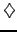[ SLIDE portfolio - no random - no arbitrage - Black-Scholes - properties || VIDEO modem - LAN - DSL]

Take a log-normal distribution of the price increments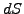in (3.3.1#eq.1) modeling the price evolution of a share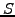with a volatility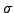in an efficient market where all the risk-free portfolios earn the same deterministic spot rate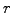. For simplicity, neglect the payment of dividends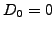and assume that the underlying trades continuously without transaction costs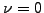(extensions are considered in exercises 3.03 & 3.04).
Now construct a portfolio combining an option (or indeed any kind of stock market derivative) with a value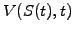and an unspecified, but constant number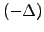of the underlying share. The initial value of this portfolio and its incremental change per time-step are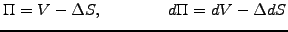(3.4#eq.1)

Using Itô's lemma (3.3.2#eq.2) to substitute for the stochastic value of the option price increment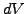and choosing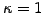for a log-normal walk with (3.3.1#eq.1), this yields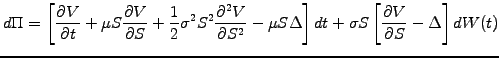(3.4#eq.2)

The random term in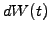can now be eliminated by continuously hedging the portfolio so as to maintain the number of shares equal to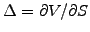. With this choice, the portfolio becomes deterministic (risk-free) and, using the standard no-arbitrage arguments, must earn the same amount as the risk-free return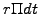payed when an amount of cash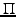is invested at a spot rateduring a small time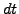. Equating both earnings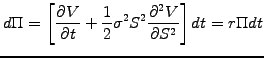(3.4#eq.3)

leads directly to the celebrated Black-Scholes equation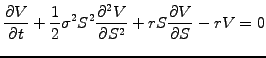(3.4#eq.4)

which shows that the return of a delta-hedged portfolio (first two term) is exactly balanced by the return on a bank deposit (last two terms). The equation describes the price of stock options (call, put and indeed any derivative security where the price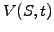depends only on the spot price and time) in a market that is parametrized by the volatilityand the spot rate. An important finding is that the price of an option does not explicitly depend on how rapidly the underlying asset grows: the drift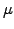does not explicitly appear in the Black-Scholes equation. The reason is that the option price has been calculated relative to the underlying, so that the drift is here already accounted for in an implicit manner.
In mathematics (3.4#eq.4) is known as a partial differential equation (since it has partial derivatives up to first order in time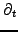and second order in the asset price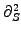) of backward parabolic type (backward because both these derivatives have the same sign, parabolic since this combination of coefficients yields exactly one characteristic). In physics, it bears a strong resemblance with the heat equation, except that here it is the price of an option that is diffusing with respect to the underlying rather than heat diffusing in space.
To solve the Black-Scholes equation backward in time, a final condition needs to be imposed at expiry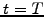reproducing the terminal payoffdiscussed in chapter 2. Boundary conditions have to be imposed on the price boundaries and are obtained from additional no-arbitrage arguments. A vanilla put option, for example, is increasingly unlikely to be exercised when the price of the underlying gets very large, so that the value of a put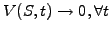when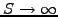. On the lower boundary, (3.3.1#eq.1) shows that the log-normal price increments of the underlying vanish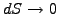when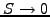, so that the problem becomes deterministic: a put option that is certain to be exercised for a price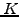at the expiry can be discounted back to the present value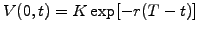. Similar arguments will be used to derive the conditions for a call option in section 4.4.

SYLLABUS  Previous: 3.3.3 Evaluate an expectancy  Up: 3 FORECASTING WITH UNCERTAINTY  Next: 3.5 Hedging a bond